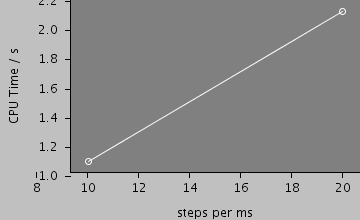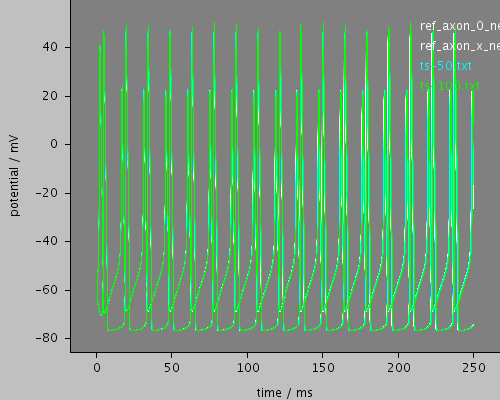rallpack3 (run.xml)

Cable with HH sodium and potassium channels, different timesteps

Uniform unbranched cable with Hodgkin-Huxley sodium and potassium channels showing a prpoagating action potential. The task specification and reference results come from Upi Bhalla's 1992 Rallpack distribution, part of which is included below. The rest in the file README.upi.

The model produces three graphs showing the reference data computed by Neuron (green) and Genesis (orange) and calculated curves in other colors according to the timestep used: magenta : 1ms, cyan : 20us, blue : 1us. The only difference between the graphs are the axis ranges: they all show the same data. The reference data was computed with 1us timesteps.

If the system performs correctly, the blue line (1us timestep) and reference green and orange should be almost coincident at the beginning and differ by less than 0.2ms after 250ms.

The magenta and cyan lines show how increasing the timestep makes very little change to the spike shape, but changes the period slightly such that by the end of the calculation the longer timestep calculation has spikes a few milliseconds behind. This is a normal feature of the implicit euler method arising from the use of forward differencing for the time derivative.

Total CPU time 3.140 seconds; at 10:35:57 Thu 24 Sep 2009

 Compartments Stochasticchannels / cpmts Continuouschannels / cpmts Non Gatedchannels / cpmts time/ms timeStep CPU Time / s 1001 0 / 0 245167 / 1001 78579 / 1001 250.0 50 2.06 1001 0 / 0 245167 / 1001 78579 / 1001 250.0 100 1.08Morphology: cellPredefined views

wholestartendAll files

 Model Preprocessed Outupt data Reference data etc run.xml cell.xml membrane.xml environment.xml recording.xml leak.xml HH_Na.xml HH_K.xml ts-50.ppp ts-100.ppp log.txt ts-50.sum ts-100.sum ts-50.dat ts-50.txt ts-100.dat ts-100.txt ref_axon_0_neuron.txt ref_axon_x_neuron.txt README README.upi

Model

Archive file of the complete model: rallpack3.jar

run.xml

<PSICSRun  timeStep="0.1ms" runTime="250ms" startPotential="-65mV"
morphology="cell"
environment="environment"
properties="membrane"
access="recording"
stochThreshold="0">
<StructureDiscretization baseElementSize="1um"/>

<info>Cable with HH sodium and potassium channels, different timesteps</info>

<RunSet vary="timeStep" values="[50, 100]us"  filepattern="ts-\$"/>

<ViewConfig>
<LineGraph width="500" height="400">
<XAxis label="time / ms"/>
<YAxis label="potential / mV"/>

<Line file="ref_axon_0_neuron.txt" color="white" width="1" rescale="[1000, 1000.]"/>
<Line file="ref_axon_x_neuron.txt" color="white" width="1" rescale="[1000, 1000.]"/>

<LineSet file="ts-10.txt" color="red"/>
<LineSet file="ts-20.txt" color="blue"/>
<LineSet file="ts-50.txt" color="cyan"/>
<LineSet file="ts-100.txt" color="green"/>

<View id="whole"/>
<View id="start"   xmin="0." xmax="30." ymin="-100." ymax="80."/>
<View id="end"   xmin="210." xmax="255." ymin="-100." ymax="80."/>

</LineGraph>
</ViewConfig>

</PSICSRun>

cell.xml

<CellMorphology id="cell">
<Point id="p0" x="0" y="0" z="0" r="0.5"/>
<Point parent="p0" id="p1" x="1000" y="0" z = "0" r="0.5"/>
</CellMorphology>

membrane.xml

<CellProperties id="membrane"
cytoplasmResistivity="100ohm_cm"
membraneCapacitance="1uF_per_cm2">

<ChannelPopulation channel="leak" density="25per_um2"/>

<ChannelPopulation channel="HH_Na" density="60per_um2"/>
<ChannelPopulation channel="HH_K" density="18per_um2"/>

</CellProperties>

environment.xml

<CellEnvironment id="environment">
<Ion id="LEAK" name="Non-specific leak" reversalPotential="-65mV"/>
<Ion id="K" name="Potassium" reversalPotential="-77mV"/>
<Ion id="Na" name="Sodium" reversalPotential="50mV"/>
</CellEnvironment>

recording.xml

<Access id="recording" recordClamps="false">

<CurrentClamp label="start" at="p0" lineColor="red" hold="0.1nA" record="true">

</CurrentClamp>

<VoltageRecorder label="end" at="p1" lineColor="blue"/>

</Access>

leak.xml

<KSChannel id="leak" gSingle="0.01pS" permeantIon="LEAK">
<OpenState id="o1"/>
</KSChannel>

HH_Na.xml

<KSChannel id="HH_Na" permeantIon="Na" gSingle="20pS">

<KSComplex id="m" instances="3">
<ClosedState id="c"/>
<OpenState id="o"/>
<ExpLinearTransition from="c" to="o" rate="1.per_ms" midpoint ="-40.mV" scale="10mV"/>
<ExpTransition from="o" to = "c" rate="4.per_ms" midpoint="-65.mV" scale="-18mV"/>
</KSComplex>

<KSComplex id="h">
<ClosedState id="c"/>
<OpenState id="o"/>
<ExpTransition from="c" to="o" rate="0.07per_ms" midpoint="-65.mV" scale="-20.mV"/>
<SigmoidTransition from="o" to="c" rate="1per_ms" midpoint="-35mV" scale="10mV"/>
</KSComplex>

</KSChannel>

HH_K.xml

<KSChannel id="HH_K" permeantIon="K" gSingle="20pS">

<KSComplex id="n" instances="4">
<ClosedState id="c"/>
<OpenState id="o"/>
<ExpLinearTransition from="c" to="o" rate="0.1per_ms" midpoint ="-55.mV" scale="10mV"/>
<ExpTransition from="o" to = "c" rate="0.125per_ms" midpoint="-65.mV" scale="-80mV"/>
</KSComplex>

</KSChannel>